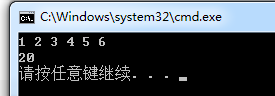3个回答

`````` int arr;
int ** p = arr;
arr = 2;
``````

``````#include<stdio.h>
int main()
{
int arr = {1, 2, 3, 4, 5, 6};
int *p;
p = arr;
p = arr;
p = arr;
printf("%d %d %d %d %d %d\n", p, p, p, p, p, p);
p = 20;
printf("%d\n", arr);
}
``````